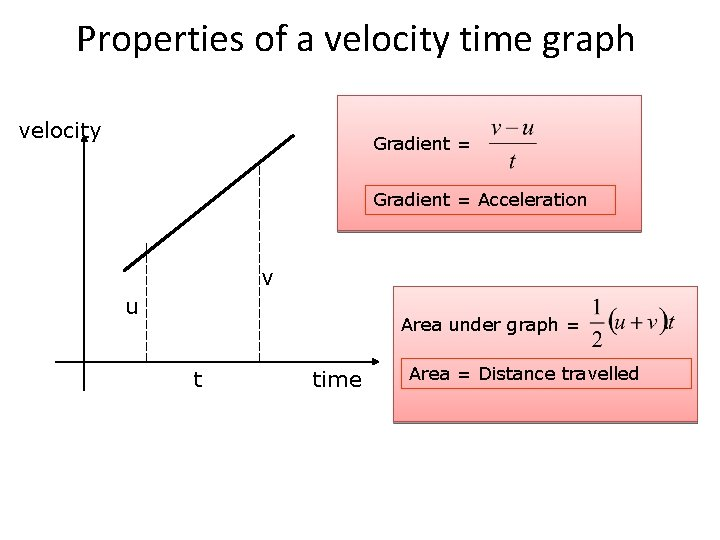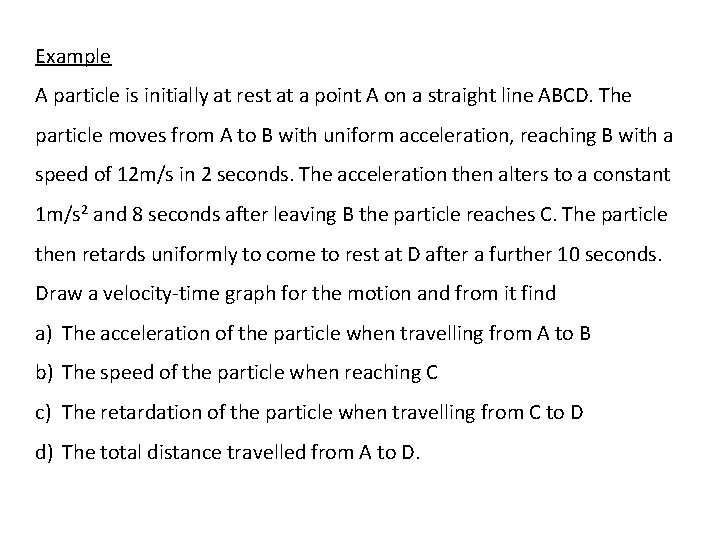# Properties of a velocity time graph velocity Gradient

• Slides: 5
Download presentationProperties of a velocity time graph velocity Gradient = Acceleration v u Area under graph = t time Area = Distance travelledExample 7 6 5 4 3 2 1 0 1 2 3 4 5 6 7 8 9 10 11 The velocity-time graph shown is for a body which starts from rest, accelerates uniformly to a velocity of 6 m/s in 2 seconds. The body then maintains this velocity for a further 6 seconds before it retards uniformly to rest. If the entire journey takes 11 seconds, find a)The acceleration of the body during the first stage of the journey b)The retardation of the body during the last stage of the journey c)The total distance travelled by the body.Example A particle is initially at rest at a point A on a straight line ABCD. The particle moves from A to B with uniform acceleration, reaching B with a speed of 12 m/s in 2 seconds. The acceleration then alters to a constant 1 m/s 2 and 8 seconds after leaving B the particle reaches C. The particle then retards uniformly to come to rest at D after a further 10 seconds. Draw a velocity-time graph for the motion and from it find a) The acceleration of the particle when travelling from A to B b) The speed of the particle when reaching C c) The retardation of the particle when travelling from C to D d) The total distance travelled from A to D.Example A train travels along a straight piece of track between two stations A and B. The train starts from rest at A and accelerates at 1. 25 ms-2 until it reaches a speed of 20 ms-1. It then travels at this steady speed for a distance of 1. 56 km and then decelerates at 2 ms-2 to come to rest at B. Find a) The distance from A to B b) The total time taken for the journey c) The average speed for the journey.A level Past paper question A train, travelling along a straight horizontal track, has a steady speed of 18 m/s as it passes the point A. Fifteen seconds later, it begins to slow down at a uniform rate for 30 s until its speed is 10 m/s. The train then increases its speed for 45 s until it reaches a speed of 20 m/s as it passes the point B. a) Draw a sketch of the v-t graph for the motion of the train between A and B. b) Calculate the acceleration of the train just before it reaches B. c) Find the distance from A to B.